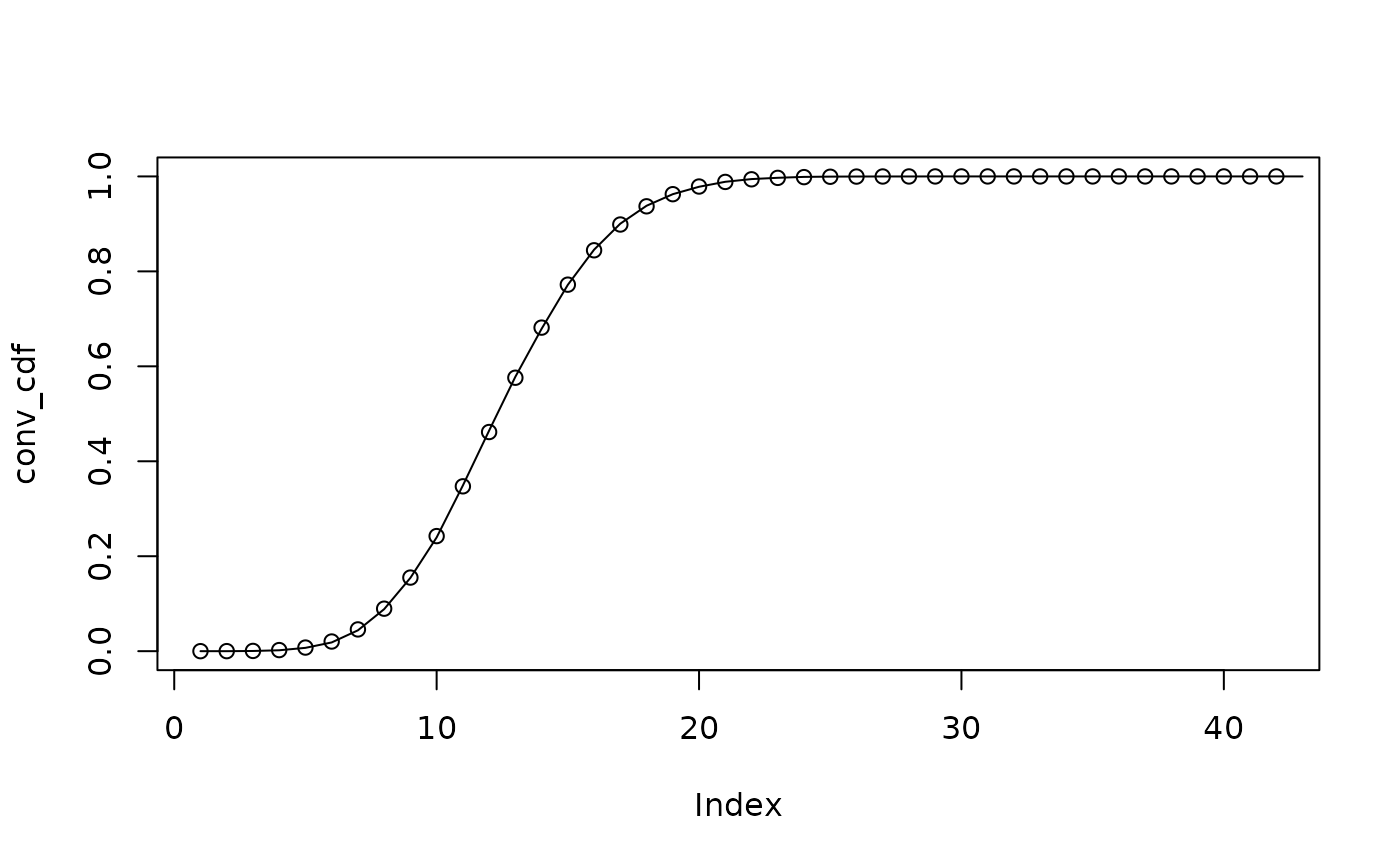This function allows the addition of probability mass functions (PMFs) to produce a new PMF. This is useful for example in the context of reporting delays where the PMF of the sum of two Poisson distributions is the convolution of the PMFs.

## Usage

add_pmfs(pmfs)

## Arguments

pmfs

A list of vectors describing the probability mass functions to

## Value

A vector describing the probability mass function of the sum of the

Helper functions for model modules convolution_matrix(), enw_reference_by_report(), enw_reps_with_complete_refs(), extract_sparse_matrix(), latest_obs_as_matrix()

## Examples

# Sample and analytical PMFs for two Poisson distributions
x <- rpois(10000, 5)
xpmf <- dpois(0:20, 5)
y <- rpois(10000, 7)
ypmf <- dpois(0:20, 7)
# Add sampled Poisson distributions up to get combined distribution
z <- x + y
# Analytical convolution of PMFs